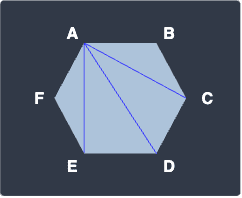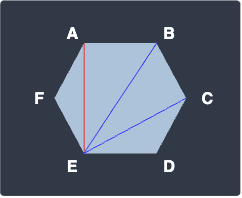search
Search
Map of Data Science
Guest 0reps
exit_to_appLog out
Map of data science
Thanks for the thanks!
close
account_circle
Profile
exit_to_app
Sign out
search
keyboard_voice
close
Searching Tips
Search for a recipe:
"Creating a table in MySQL"
Search for an API documentation: "@append"
Search for code: "!dataframe"
Apply a tag filter: "#python"
Useful Shortcuts
/ to open search panel
Esc to close search panel
to navigate between search results
d to clear all current filters
Enter to expand content previewDoc SearchCode Search BetaSORRY NOTHING FOUND!
mic
Start speaking...Voice search is only supported in Safari and Chrome.
Shrink
Navigate to
A
A
brightness_medium
share
arrow_backShareTwitterFacebook
check_circle
Mark as learned
thumb_up
0
thumb_down
0
chat_bubble_outline
0
auto_stories new
settings

# Comprehensive Guide on Combinations

Probability and Statistics
chevron_right
Probability Theory
schedule Oct 31, 2022
Last updated
local_offer Probability and Statistics
Tags
map
Check out the interactive map of data science

Before we go through the mathematical definition of combinations, let's start with a motivating example.

Example.

# Number of possible combinations of choosing people

Suppose we must choose a group of $3$ out of a total of $5$ people. How many possible combinations are there?

Solution. Let's illustrate the scenario:Here, we're referring to each person by their letter. One possible choice for the group is $\mathrm{ABC}$ and another is $\mathrm{ABD}$. However, we must make sure we don't double count, that is, if we've already counted the group $\mathrm{ABC}$, then we should not count the group $\mathrm{BAC}$ as another combination. This should make sense because groups $\mathrm{ABC}$ and $\mathrm{BAC}$ are identical - both groups have the same people in them. In fact, the word "combinations" in mathematics specifically means that the ordering of the elements within a group does not matter.

How should we go about counting all possible combinations without double counting? Our plan of attack is to count all possible permutations first and then remove the double counts.

Recall from our guide on permutations that the total number of ways to select a group of $3$ from $5$ people is:

$$\begin{equation}\label{eq:wyoLmDM1wGk34IgoBTV} {\color{red}\frac{5!}{2!}}=5\times4\times3=60 \end{equation}$$

The problem is that this number includes double counts. Our goal now is to discard these double counts. The key is to think about how many times we are counting each group. For instance, consider the group $\mathrm{ABC}$ - let's list all the permutations of $\mathrm{ABC}$ below:

 ABC, ACB, BAC, BCA, CAB, CBA 

We can use permutations once again to compute the number of arrangements, that is, $3!=6$. Because each group is counted $6$ times and we have $60$ groups (with duplicates), the total number of possible combinations (without duplicates) is:

$$\begin{equation}\label{eq:BlPvnZbjzvbGB7zIW9z} {\color{red}\frac{5!}{2!}}\times\frac{1}{3!}=60\times\frac{1}{3!}=10 \end{equation}$$

Let's generalize this technique of counting combinations. Let $n$ be the number of distinct elements (e.g. people) and $r$ be the number of slots to fill. In our example, $n=5$ and $r=3$. The count of ordered elements is computed by permutation as:

$$\begin{equation}\label{eq:rGaLX4K49ircyGf1BJO} \frac{n!}{(n-r)!} \end{equation}$$

If we plug in $n=5$ and $r=3$ as in our example, we would get \eqref{eq:wyoLmDM1wGk34IgoBTV}.

Next, we remove double counts by dividing \eqref{eq:rGaLX4K49ircyGf1BJO} by $r!$, which represents the number of ways to arrange any group:

$$\begin{equation}\label{eq:Rti5pFOMLFOeFInZDMZ} \frac{n!}{r!(n-r)!} \end{equation}$$

Again, if we substitute $n=5$ and $r=3$ as in our example, we would get \eqref{eq:BlPvnZbjzvbGB7zIW9z}. What we have managed to derive here is the formula for combinations!

Definition.

# Definition and formula for combinations

Combination refers to the number of ways of selecting $r$ elements from a total of $n$ elements without regard to order:

$${n\choose{r}}=\frac{n!}{r!(n-r)!}$$

The left side is read as "n choose r".

# Difference between permutations and combinations

The main difference between permutations and combinations is that permutations are concerned with the ordering of the chosen elements whereas combinations are not.

For instance, the number of permutations of selecting two distinct letters from $\mathrm{A}$, $\mathrm{B}$ and $\mathrm{C}$ is:

$$_3P_2=\frac{3!}{(3-2)!}=6$$

On the other hand, the number of combinations for the same task is:

$${3\choose{2}} =\frac{3!}{2!\times(3-2)!}=3$$

The formulas for permutations and combinations look similar, and in fact, combinations can be computed from permutations:

$${3\choose{2}} \;=\;_3P_2\times\frac{1}{2!}=3$$

As we can see, computing combinations involves an additional step of removing double counts by dividing by the number of arrangements of any selection ($2!$ in this case). This also means that permutations must always be equal to greater than combinations for the same task.

Let's now go through some examples of applying the formula for combinations!

# Examples

Example.

## Number of combinations drawing balls

Suppose we have $8$ balls in a bag, of which $3$ are red and $5$ are green. How many possible combinations are there for choosing $2$ red and $4$ green balls?

Solution. The number of combinations of choosing $2$ red balls from $3$ red balls is:

\begin{align*} {3\choose{2}} &=\frac{3!}{2!\times(3-2)!}\\ &=3 \end{align*}

Here, the ordering of the red balls does not matter. For instance, if we label the balls as $\color{red}\mathrm{R}_1$, $\color{red}\mathrm{R}_2$ and $\color{red}\mathrm{R}_3$, then $\color{red}\mathrm{R}_1\color{red}\mathrm{R}_2$ is the same as $\color{red}\mathrm{R}_2\color{red}\mathrm{R}_1$. This is the reason why we use combinations instead of permutations here.

The number of combinations of choosing $4$ green balls from $5$ green balls is:

\begin{align*} {5\choose{4}} &=\frac{5!}{4!\times(5-4)!}\\ &=5 \end{align*}

The total possible number of combinations is their product, that is, $3\times5=15$.

Example.

## Number of diagonals of a hexagon (optional)

How many diagonals does a hexagon have?

Solution. Each vertex of a hexagon has $3$ diagonals:Since a hexagon has $6$ vertices, the total number of diagonals is:

$$6\times3=18$$

However, this number is misleading because we are double counting the diagonals. For instance, the diagonals from vertex $\mathrm{E}$ are:Here, the diagonal $\mathrm{AE}$ is counted once again. Therefore, even though there are $18$ ordered diagonals, half of them are duplicated. Therefore the total number of unique diagonals is:

$$\frac{18}{2}=9$$

Even though this question does not use the combinations formula, the main idea is the same - we first compute the total number of permutations and then remove duplicates by division.